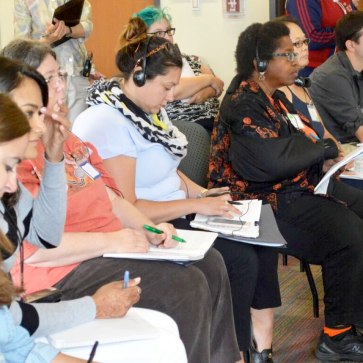# Teaching Through Problem-solving (TTP)

This course provides a structured, in-depth look at Teaching Through Problem-Solving, an approach that builds students’ mathematical knowledge, reasoning, and dispositions through problem-solving.

##### HOURS:

10-12 hoursModule 1 | Introduction to TTP

In this module, you will:

• Be introduced to TTP, and learn how it supports students to learn mathematics
• Consider how TTP relates to the curriculum
• Learn about the four phases of a TTP lesson

## 1.1: Teaching Through Problem-Solving

Teaching Through Problem-Solving (TTP) is a mathematics teaching approach that is widespread in Japan. Students develop each new mathematical concept in the curriculum by solving a problem that illuminates the concept.  These are truly “problems” as mathematicians define them–and not exercises–because students have not previously learned a procedure or formula to solve the problem. As students draw on prior knowledge to solve these novel problems, they constantly build and use mathematical practices and reasoning.  So mathematical practices and reasoning are seamlessly integrated within daily instruction.

Teaching Through Problem-Solving (mondai kaiketsu gakushu) helped inspire the widely-known 5 Practices for Orchestrating Productive Mathematics Discussions.

Watch the short video below showing excerpts of a Teaching Through Problem-solving lesson with teacher and student reflections.

#### Why Teaching Through Problem-Solving?

Teaching Through Problem-Solving helps students to:

• See mathematics as a coherent body of ideas they themselves develop using their prior knowledge
• Become perseverant mathematics learners who expect to confront novel problems they have never been taught to solve
• Build mathematical agency as they see their thinking and writing influence classmates’ learning

#### Reflection Questions

1. How do you currently use problem-solving in your instruction?
2. How do you typically introduce new mathematical concepts and procedures?
3. How confident are your students when confronted with problems they have not previously been taught to solve?

## 1.2: How Teaching Through Problem-Solving Relates to the Curriculum

In Teaching Through Problem-solving, students use their current knowledge to build the next big mathematical concept or procedure in the curriculum.

To achieve this goal, teachers need to think deeply about:

• the curriculum
• what students know
• how students can use their current knowledge to build the next idea in the curriculum–rather than just be told it

Some curriculum materials make it easy to see how students can use their prior knowledge to build the next concept, whereas other curriculum materials may require more careful study. Mathematical progressions and standards can also provide important insights into how students build their knowledge of new content. The examples in this course illustrate how students build knowledge, and should help you bring this vision of student learning to your own curriculum.

#### Reflection Questions

1. Do the curriculum materials you use make it easy to see how students can use their prior knowledge to build the next concept?  If so, find an example and explain how they do this.

## 1.3: The 4 Phases of a TTP Lesson

Click on the flowchart below to read a summary of each phase and click on a video example to see what that phase looks like at grade K, 2 and 5.

Grasp the Problem

#### WHAT STUDENTS DO

Understand the problem and develop interest in solving it.

Consider what they know that might help them solve the problem.

#### WHAT TEACHERS DO

Show several student journal reflections from the prior lesson.

Pose a problem that students do not yet know how to solve.

Interest students in the problem and in thinking about their own related knowledge.

Try to Solve

#### WHAT STUDENTS DO

Independently try to solve the problem.

Do not simply follow the teacher’s solution example.

Allow classmates to provide input after some independent work time.

#### WHAT TEACHERS DO

Circulate, using seating chart to note each student’s solution approach.

Identify work to be presented and discussed at board.

Ask individual questions to spark more thinking if some students finish quickly or don’t get started.

#### VIDEO

Present & Discuss

#### WHAT STUDENTS DO

Present and explain solution ideas at the board, are questioned by classmates and teacher. (2-3 students per lesson)

Actively make sense of the presented work and draw out key mathematical points. (All students)

#### WHAT TEACHERS DO

Strategically plan sequence of student presentations of work at the board, to build the new mathematics. (Incorrect approaches may be included.)

Monitor student discussion: Are all students noticing the important mathematical ideas?

Add teacher moves (questions, turn-and-talk, votes) as needed to build important mathematics.

#### VIDEO

Summarize & Reflect

#### WHAT STUDENTS DO

Consider what they learned and share their thoughts, to help formulate class summary of learning. Copy summary into journal.

Write journal reflection on their own learning from the lesson.

#### WHAT TEACHERS DO

Write on the board a brief summary of what the class learned during the lesson, using student ideas and words where possible.

Ask students to write in their journals about what they learned during the lesson.

#### VIDEO

New Learning

The New Learning is what students actually take away from the lesson, as reflected in their journal writing, their work and ideas during class, and the exit task, if any. Identifying what students took away, and noticing any ideas that are fragile or partial, allows teachers to plan the next lesson.

#### Reflection Questions

1. How are the phases of Teaching Through Problem-Solving similar to or different from your current instruction?
2. What intrigues you?
3. What do you expect to be most challenging about using a Teaching Through Problem-solving approach in your classroom?